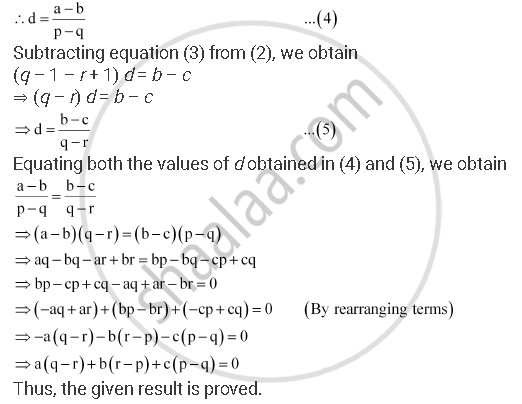CBSE (Arts) Class 11CBSE
Share
Notifications

View all notifications
Books Shortlist
Your shortlist is empty

# The Pth, Qth And Rth Terms of an A.P. Are A, B, C Respectively. Show That (Q – R )A + (R – P )B + (P – Q )C = 0 - CBSE (Arts) Class 11 - Mathematics

Login
Create free account

Forgot password?
ConceptArithmetic Progression (A.P.)

#### Question

The pthqth and rth terms of an A.P. are a, b, c respectively. Show that (q – r )a + (r – p )b + (p – q )c = 0

#### Solution

Let t and d be the first term and the common difference of the A.P. respectively.

The nth term of an A.P. is given by, an + (n – 1d

Therefore,

ap = t + (p – 1d = a … (1)

aq = t + (q – 1)d = b … (2)

ar = t + (r – 1d = c … (3)

Subtracting equation (2) from (1), we obtain

(p – 1 – q + 1) d = a – b

⇒ (p – qd = a – bIs there an error in this question or solution?

#### APPEARS IN

NCERT Solution for Mathematics Textbook for Class 11 (2018 to Current)
Chapter 9: Sequences and Series
Q: 15 | Page no. 199
Solution The Pth, Qth And Rth Terms of an A.P. Are A, B, C Respectively. Show That (Q – R )A + (R – P )B + (P – Q )C = 0 Concept: Arithmetic Progression (A.P.).
S